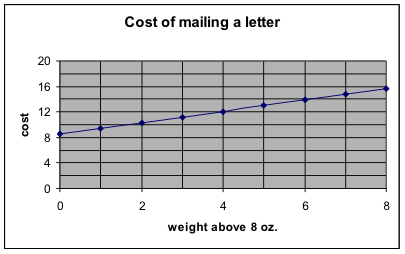## Alternative method of solution for Example 3:

Example 3 question: For a certain type of letter sent by Federal Express, the charge is $8.50 for the first 8 ounces and$0.90 for each additional ounce (up to 16 ounces.)   How much will it cost to send a 12-ounce letter?

We saw here that it is awkward for the value to be so far from any useful values for the variables. This suggests that we re-define the variables to be more useful.

1-2. Define the variables and their units and possible values. List the points.

Let x = number of ounces above 8. So the possible values for x are 0 to 8 (which corresponds to the letter weighing 8 ounces to 16 ounces.)

Let y = cost. The possible values for y are $8.50 and larger. A letter weighing 8 ounces costs$8.50. So x = 0. The point is (0, 8.50)

A letter weighing 9 ounces costs $8.50+0.90 =$9.40.   So x = 1. The point is (1, 9.40)

3. Is a linear model appropriate?

Yes, because the cost increases by the same amount for each additional ounce.

4-5. Find the slope and the formula.

Using the points above, we have

6. Interpret the y-intercept.

When x = 0, the value for y is 8.50. Since x = weight – 8 ounces, that means that when the letter weighs 8 ounces, the cost is $8.50. Notice that defining the x-variable in this manner has made the y-intercept more meaningful in the problem. 7. Make the prediction. If the letter weighs 12 ounces, then we use x = 12 – 8 = 4. So $y=8.50+0.90\cdot4=8.50+3.60=12.10$. This agrees with the answer we found by the first method. 8. Graph and check whether you obtain the same results.  weight x = weight – 8 y=cost 8 0 8.5 9 1 9.4 By hand, plot these points, draw the line, extend it to the limits for the variables, and use it to estimate the answer to the question.On the graph, look up a weight of 12 ounces, which is x = 12 – 8 = 4. The cost for x = 4 is a little larger than$12, which is consistent with the value we obtained from the formula.